Introduction to Hydrology (Geog 3511)

Fall 2012

Assignment 8: Material Properties of Soil Water in the Unsaturated Zone

 Section Lab Assigned Due 012,013 Tuesday Tuesday, Oct 30 Friday, Nov 2 011 Thursday Thursday, Nov 1 Monday, Nov 5

40 possible points.

CITE SOURCES OF EQUATIONS USED.

WRITE THE QUESTION AT THE START OF EACH ANSWER.

### Material Properties of Soils (6 Points)

We have collected a soil sample using a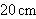long cylindrical tube having a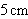diameter. Wet weight of the soil sample was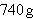. After oven-drying at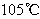the sample weighed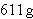. Assume the particle density () was.

Q1) What was the bulk density?

Q2) What was the porosity?

Q3) What was the water content?

Q4) What was the wetness (or degree of saturation)?

### Pressure Head and Volumetric Moisture Content (7 points)

Use the following link to download pressure head and volumetric moisture content data obtained from a fine sand. Data like this are typically obtained from laboratory experiments.

Q5) Graph pressure head (meters of water; x-axis) versus volumetric moisture content (fraction; y-axis).

Q6) At what volumetric water content does the soil wetness (S) equal 1? How did you figure this out?

Q7) What is the height of the capillary fringe? How did you figure this out?

Q8) Is the volumetric water content of 0.05 less than, equal to, or greater than the permanent wilting point? How did you figure this out?

### Volumetric Moisture Content and Hydraulic Conductivity (8 points)

Q9) Graph volumetric moisture content (x-axis) versus hydraulic conductivity (y-axis), using this data from a fine sand. Use a linear-log plot, with hydraulic conductivity (y-axis) plotted on a log scale.

Q10) How does the hydraulic conductivity change with increasing water content?

Q11) What is the hydraulic conductivity at Ksat?

Q12) What is the hydraulic conductivity at a pressure head of -0.35 m?

Q13) What is the hydraulic conductivity at a pressure head of -0.55 m?

### Darcy's Law (19 points)

Tensiometers are installed at 0.4 m and 0.5 m above the water table in a uniform sandy soil with the moisture characteristic and hydraulic conductivity curves from the two problems above. The tensiometer readings are -0.45 m at 0.4 m above the water table and -0.6 m at 0.5 m above the water table. Typical values of hydraulic conductivity can be found in Dingman Table 6-1.

Q14) What is Darcy's Law? Give the equation and an interpretation of each parameter. What is Darcy's law used for?

Q15) What is the hydraulic gradient (estimated from the tensiometer measurements)?

Q16) Is the water movement up (towards the surface) or down? Include your reasoning.

Q17) What is the moisture content at the 0.4 tensiometer?

Q18) What is the moisture content at the 0.5 tensiometer?

Q19) What is the hydraulic conductivity at the 0.4 tensiometer?

Q20) What is the hydraulic conductivity at the 0.5 tensiometer?

Q21) Calculate the specific discharge at the 0.4 tensiometer.

Q22) Calculate the specific discharge at the 0.5 tensiometer.